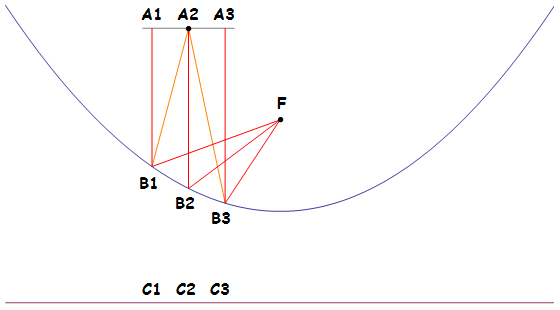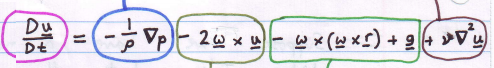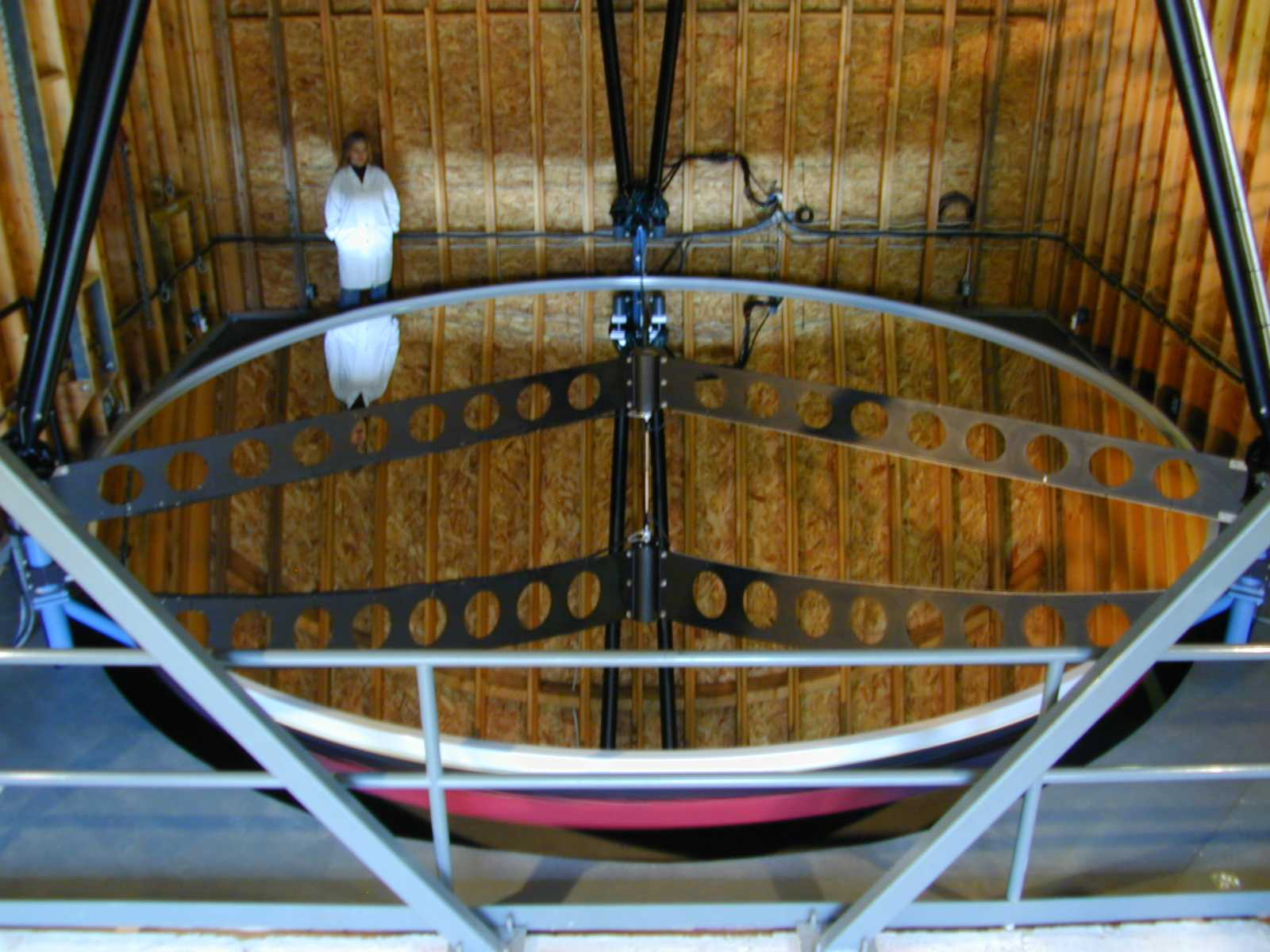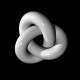# Liquid mirror telescopes

Breaking news: Following the selection process at Oundle, the UK IMO team has now been selected. It comprises Gabriel Gendler, Frank Han, Freddie Illingworth, Warren Li, Harvey Yau and Joe Benton. It is very worrying that two of these people belonged to the same Balkan Mathematical Olympiad team responsible for a non-LaTeXed report!

Anyway, a particularly useful mathematical coincidence, first observed by Newton, is that the shape assumed by the surface of a rotating container of liquid is precisely the shape necessary to focus parallel light to a single point. It is a rather elementary exercise to prove this, and amazingly does not require the use of calculus.

Theorem 1 (Archimedes): Light incident on a parabola from a direction perpendicular to its directrix is reflected to the focus.

Proof: Consider a light beam originating at A2 and travelling to F in the following diagram. We wish to show that the path A2 — B2 — F is shorter than any of the alternative paths, such as A2 — B1 — F and A2 — B3 — F, as then we would win by Fermat’s principle that light travels from source to destination in least* time over all such paths.Recall that a parabola is defined to be the set of points that are equidistant from the focus and directrix. In other words, d(F, B1) = d(B1, C1), d(F, B2) = d(B2, C2), and d(F, B3) = d(B3, C3), where d(X, Y) denotes the distance between points X and Y. Also, observe that d(A1, C1) = d(A2, C2) = d(A3, C3), since these are just parallel lines of the same length. Consequently, we obtain the following result:

• The red rays are all of the same length.

Together with the fact that d(A2, B1) > d(A1, B1) and d(A2, B3) > d(A3, B3), this means that the path A2 — B2 — F is indeed shorter than the alternative paths. Q. E. D.

* Technically, it follows a path such that the time functional is stationary with respect to small variations in the path. This may be a maximum or saddle point rather than a (local) minimum, but this is rare.

Corollary 2: Light perpendicular to the directrix incident upon a paraboloid is reflected to the focus.

Proof: Just take the two-dimensional slice containing the ray of light and the focus, then apply Theorem 1.This proof is legitimate, as we know that the light ray must remain in this plane by the symmetry of the configuration.

Corollary 3 (Newton): It is possible to build a telescope using a paraboloid mirror.

Proof: You can buy one from Celestron.

Theorem 4 (Newton): Neglecting surface tension, the surface of a fluid in a container rotating at uniform angular speed about a vertical axis assumes the shape of a paraboloid.

Proof: Operate in the reference frame of the rotating container. Due to fluid friction (viscosity), the fluid will eventually become stationary, so u = 0 everywhere. Recall that the Navier-Stokes equation is:Since u = 0, most things vanish and we are left with −∇p/ρ + ω²r er − g ez = 0, where r is the distance from the axis of rotation and g is the acceleration due to gravity, and the things in bold are unit vectors. The last expression can be expressed as a gradient of a scalar potential, leading to the following equation:

• ∇(p/ρ − ½ω²r² + gz) = 0

Consequently, p/ρ − ½ω²r² + gz is constant throughout the fluid, including on the surface. Moreover, p is equal to the atmospheric pressure on the surface, so − ½ω²r² + gz is constant. This is the equation of a paraboloid with focal length g/2ω².

Newton realised that it is thus possible to build a telescope by using a container of reflective fluid rotated at constant speed. Unfortunately, maintaining a constant speed of rotation was prohibitively difficult, so he settled for hand-grinding a spherical mirror instead (spheres and paraboloids agree to quadratic order, which was good enough).

Nevertheless, liquid mirror telescopes now exist, the largest being the six-metre Large Zenith Telescope (so called because it points directly upwards). This has a parabolic mirror made from liquid mercury rotating at an angular speed of ω = 0.700 to maintain a focal length of 10.00 metres.We have neglected surface tension in the derivation. Surface tension makes the surface of the liquid slightly more spherical, since a sphere has constant mean curvature whereas a paraboloid does not. The contribution from surface tension, however, is so minuscule that this doesn’t matter whatsoever.

A more important drawback is that the mirror can only point upwards, in the opposite direction to gravity. There are at least three ways to circumvent this:

• Use a space telescope with artificial gravity induced by rotation about another axis. No explicit examples have been constructed.
• Allow the liquid to solidify whilst being rotated. The result is a solid parabolic mirror. I was reading the new book that Alex Bellos sent me, entitled Alex Through the Looking Glass, which mentions that the Large Binocular Telescope was constructed using this method. Unfortunately, it is still required to correct the mirror by a slight amount of grinding (typically by sand-blasting with ions instead of sand).
• Use a large planar mirror, which is cheaper to construct than a curved mirror, to reflect light onto the paraboloid.
This entry was posted in Uncategorized. Bookmark the permalink.

### 4 Responses to Liquid mirror telescopes

1.Bill Gosper says:

A fourth route to telescope parabolicity:

2.Volker says:

There’s a typo at:

d(A1, C1) = d(A2, C2) + d(A3, C3)

It should be:

d(A1, C1) = d(A2, C2) = d(A3, C3)

•apgoucher says:

Thanks! Amended.

3.jair2018 says:

Reblogged this on DataWorks.